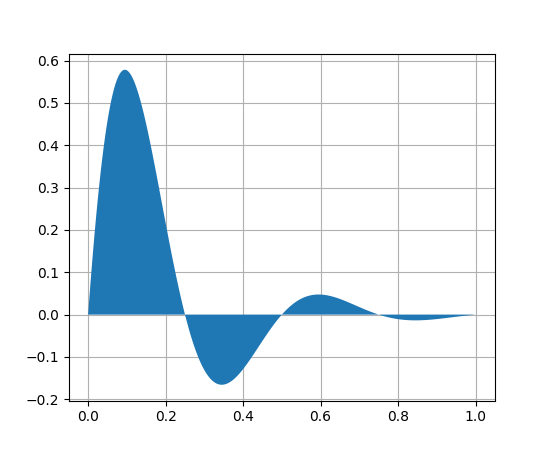Travis-CI:# matplotlib.axes.Axes.fill¶

`Axes.``fill`(*args, **kwargs)

Plot filled polygons.

Parameters: Returns: args : a variable length argument It allowing for multiple x, y pairs with an optional color format string; see `plot()` for details on the argument parsing. For example, each of the following is legal: ```ax.fill(x, y) ax.fill(x, y, "b") ax.fill(x, y, "b", x, y, "r") ``` An arbitrary number of x, y, color groups can be specified:: ax.fill(x1, y1, ‘g’, x2, y2, ‘r’) a list of `Patch` kwargs : `Polygon` properties

Notes

The same color strings that `plot()` supports are supported by the fill format string.

If you would like to fill below a curve, e.g., shade a region between 0 and y along x, use `fill_between()`

ExamplesNote

In addition to the above described arguments, this function can take a data keyword argument. If such a data argument is given, the following arguments are replaced by data[<arg>]:

• All arguments with the following names: ‘x’, ‘y’.